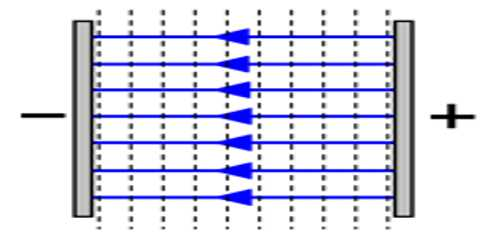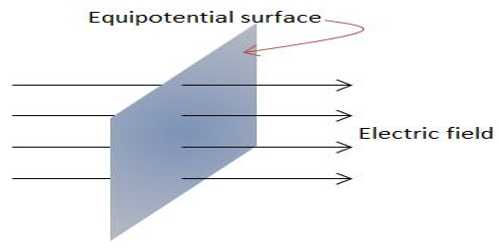# Uniform Electric Field

Uniform electric field: If the magnitude and direction of the electric field are same everywhere, then the field is called uniform electric field [Figure]. Example: A pair of parallel electric plates has a potential difference of 200 V across its plates.

A uniform electric field is set up when there are two in straight line parallel electrodes (plates) that have a potential difference between them. If a positive charge is placed at a point halfway between the plates then it will shift toward the negative electrode (electric attraction) along the electric field lines. It is represented by parallel lutes of the force of equal spacing. The field lines are parallel to each other and at right angles to the plates. They go from the positive plates and move toward the negative plates.The field lines are parallel to each other and at right angles to the plates. They go from the positive plates and move toward the negative plates. The field lines will curve around at the end of the plates.